Question
A compound is known to have a free amino group with a pKa of 8.8, and one other ionizable group with a pKa between 5 and 7. To 100 mL of a 0.2 M solution of thiscompound at pH 8.2 was added 40 mL of a solution of 0.2 M hydrochloric acid. The pH changed to 6.2. The pKa of the second ionizable group is:

We need at least 10 more requests to produce the answer.

0 / 10 have requested this problem solution

The more requests, the faster the answer.

All students who have requested the answer will be notified once they are available.

#### Earn Coins

Coins can be redeemed for fabulous gifts.

Similar Homework Help Questions
• ### calculating the pKa

A compound is known to have a free amino group with a pKa of 8.8, and one other ionizable group with a pKa between 5 and 7. To 100 mL of a 0.2 M solution of thiscompound at pH 8.2 was added 40 mL of a solution of 0.2 M hydrochloric acid. The pH changed to 6.2. The pKa of the second ionizable group is:

• ### A compound is known to have a free amino group with a pk of 8.8, and...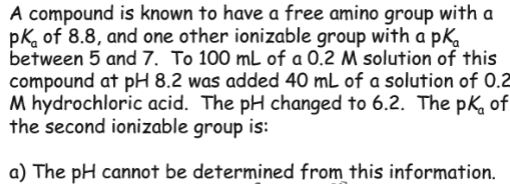A compound is known to have a free amino group with a pk of 8.8, and one other ionizable group with a pKo between 5 and 7. To 100 mL of a 0.2 M solution of this compound at pH 8.2 was added 40 mL of a solution of 0.2 M hydrochloric acid. The pH changed to 6.2. The pK, of the second ionizable group is: a) The pH cannot be determined from this information. 5566 bcde

• ### 19. Calculation of pH after Titration of Weak Acid A. compound has a pk of 7.4....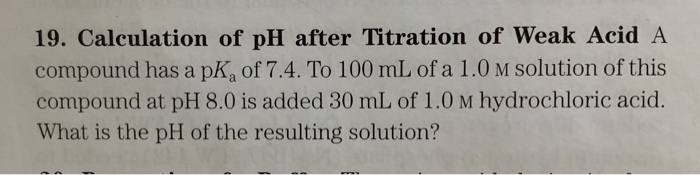19. Calculation of pH after Titration of Weak Acid A. compound has a pk of 7.4. To 100 mL of a 1.0 m solution of this compound at pH 8.0 is added 30 mL of 1.0 m hydrochloric acid. What is the pH of the resulting solution?

• ### 1. Calculation of pH. A weak acid HA (pKa = 4.85) reacted with strong base NaOH The reaction is HA + NAOH H20 + NaA...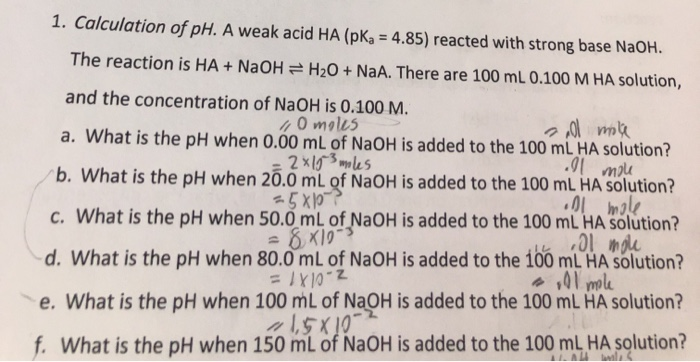1. Calculation of pH. A weak acid HA (pKa = 4.85) reacted with strong base NaOH The reaction is HA + NAOH H20 + NaA. There are 100 mL 0.100 M HA solution, and the concentration of Na OH is 0.100 M. 0 moles a. What is the pH when 0.00 mL of NaOH is added to the 100 mL HA solution? 2 x1 les b. What is the pH when 20.0 mL of NaOH is added to the 100...

• ### 20. Properties of a Buffer The amino acid glycine is often used as the main ingredient...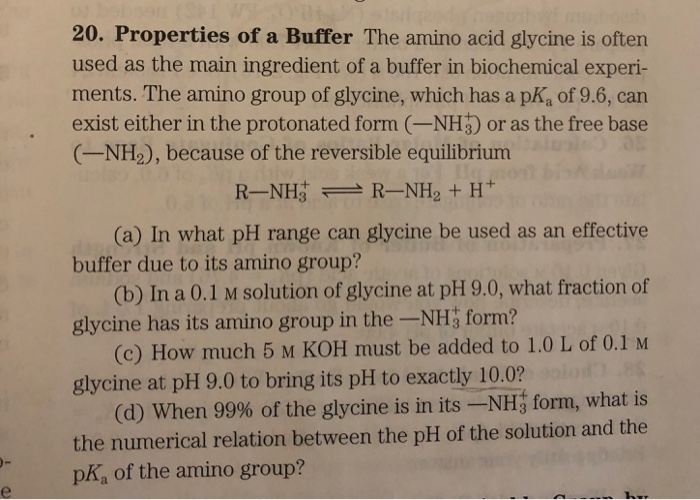20. Properties of a Buffer The amino acid glycine is often used as the main ingredient of a buffer in biochemical experi- ments. The amino group of glycine, which has a pKa of 9.6, can exist either in the protonated form (-NH3 or as the free base (-NH2), because of the reversible equilibrium (a) In what pH range can glycine be used as an effective buffer due to its amino group? (b) In a 0.1 M solution of glycine at...

• ### A buffer solution is made by adding 1.00 M weak acid (pKa = 4.45) to 1.25...

A buffer solution is made by adding 1.00 M weak acid (pKa = 4.45) to 1.25 M solution of its conjugate base to make 100 mL of buffer solution.  Calculate the pH after 75.0 mL of 0.725 M strong acid has been added.

• ### Draw the titration curve for the titration of 20 mL of a 0.12 M solution of...

Draw the titration curve for the titration of 20 mL of a 0.12 M solution of glutamate (carboxylic acid pKa = 2.19, amino pKa = 9.67, you need to know the pKa for the R group) with 0.10 M sodium hydroxide. Clearly label the axes of your graph (pH vs mL of NaOH added.) Be sure to include pH values and volumes for the following: Start of the titration (no sodium hydroxide added), the pH at the volume that is...

• ### Consider a weak base that has a pKb of 4.2. • What is the pKa of...

Consider a weak base that has a pKb of 4.2. • What is the pKa of this solution? • What is the pH of a 0.2 M solution of this base? • If you were to mix 50 mL of the 0.2 M base with 50 mL of a 0.1 M solution of its conjugate acid, what would the pH of this buffer be, and what is its effective buffering range?

• ### 6. How many mL of 0.2 M sodium acetate should be added to 200 mL of...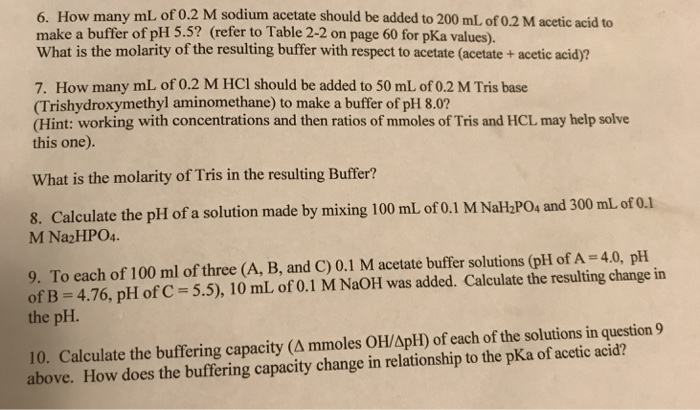6. How many mL of 0.2 M sodium acetate should be added to 200 mL of 0.2 M acetic acid to make a buffer of pH 5.5? (refer to Table 2-2 on page 60 for pKa values). What is the molarity of the resulting buffer with respect to acetate (acetate + acetic acid)? 7. How many mL of 0.2 M HCl should be added to 50 mL of 0.2 M Tris base (Trishydroxymethyl aminomethane) to make a buffer of pH...

• ### Suppose 500 mL of 0.200 M nitrous acid , which has a pKa of 3.347, solution...

Suppose 500 mL of 0.200 M nitrous acid , which has a pKa of 3.347, solution is titrated with 75.0 mL of a 1.00 M NaOH. What is the final pH? The error interval is +/- 0.2 pH units.